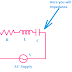The main difference between Impedance and Resistance is, Impedance is the opposition to the flow of electric current in alternating nature whereas Resistance is the opposition to the flow of electric current in any nature. So you may understand they both oppose the flow of electric current. This was the actual concept. Now, let's go to make a comparison between them in a detailed explanation that will help you to understand all the concepts. Impedance and Resistance both are very important and well-known terms in basic electrical engineering. So, having a very clear knowledge of them will help you to understand all the complex topics related to them.

## What is Impedance? It's Meaning

Impedance means something that impedes or interferes. Impedance is the property of a circuit consisting of different elements by virtue of which it opposes the flow of electric current. Impedance is the combination of resistance and reactance or standalone reactance.

Impedance is not a constant internal physical property of any component or element. It is produced due to some phenomenon of the nature of the electricity and component type. For example, when a DC current flow in an RL(Resistive-Inductive) Circuit there is no impedance occurs. There is only the resistance. But when in that same circuit an alternating current flows the impedance occurs that impedes or interferes to oppose the flow of alternating current.

Now the question is why impedance occurs when alternating current flows. The simple answer is it is happening due to the inductive reactance of the inductor. We know that the inductor does not show any reactance property when it is supplied by a DC power supply but when it is connected with an AC power supply, the inductor shows the inductive reactance property due to its self-inductance property. Same the capacitor also does not shows any reactance property with DC power supply, it shows the capacitive reactance property when AC power supply is applied to it.

## What is Resistance? It's Meaning

Resistance means something that resists or opposes. Resistance is a property of an object or material by virtue of which it resists or opposes the flow of electric current through it. So you may understand it is an internal physical property of any material or circuit component that is almost constant. For example, a resistor is an element that shows only the resistance property with both AC and DC Power supplies. It does not show any reactance property to the AC power supply.

The resistance property of an element does not change with the changing of the nature of the power supply. Resistance property opposes the flow of any type of electric current uniformly. For example, a resistor of 2 Ohm will always show the 2 Ohm resistance. The resistance property of a material is only changed by other physical quantities such as temperature, shape, sizes, types of material, etc.

Not only the resistor has the resistance property. In fact, all the physical elements such as inductors and capacitors also have their own resistance property. But it is very less. These inductors and capacitors are only shown their resistance property when they are operating with DC current but when they are supplied AC supply, they will show both resistance and reactance properties. And this combination of resistance and reactance is known as impedance.

## The key differences between Impedance and Resistance

 Impedance Resistance It is the opposition to the alternating current It is the opposition to the electric current in any nature It depends upon the resistance, reactance, and the nature of the current It depends upon the physical property of the components The SI unit of Impedance is Ohm The SI Unit of Resistance is also Ohm Impedance is denoted by the English letter ‘Z’ Resistance is denoted by the English letter ‘R’ Impedance varies with the supply frequency Resistance does not vary with the supply frequency Impedance is generally expressed in complex numbers Resistance is generally expressed in real numbers Impedance relates to Phase angle Resistance does not relate to any phase angle

Impedance VS Resistance (Difference with their actual Meaning)Reviewed by Author on 6/22/2022 Rating: 5